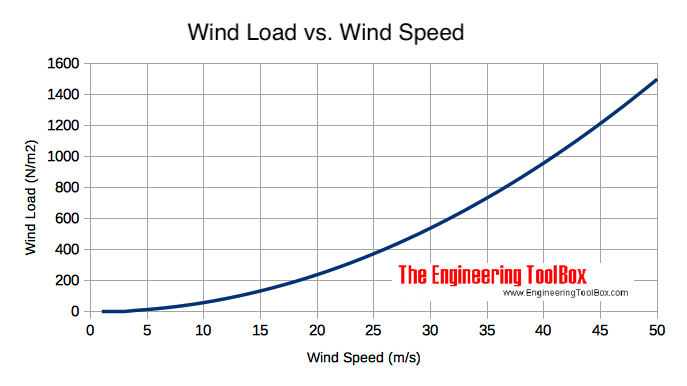Engineering ToolBox - Resources, Tools and Basic Information for Engineering and Design of Technical Applications!

# Dynamic Pressure

## Dynamic pressure is the kinetic energy per unit volume of a fluid in movement.

Dynamic pressure is the kinetic energy of a flowing fluid - liquid or gas - per unit volume - and can be expressed as

pd = 1/2 ρ v2                        (1)

where

pd = dynamic pressure (N/m2 (Pa), lbf/ft2 (psf))

ρ = density of fluid (kg/m3, slugs/ft3)

v = velocity (m/s, ft/s)

### Dynamic Pressure Calculator - SI Units

The default values below are for water with density of 1000 kg/m3.

ρ - density of fluid (kg/m3)

v - velocity (m/s)

• 1 N/m2 = 1 Pa = 1.4504x10-4 lbf/in2 (psi) = 0.02089 lbf/ft2 (psf) = 1x10-5 bar = 4.03x10-3 in water = 0.336x10-3 ft water = 0.1024 mm water = 0.295x10-3 in mercury = 7.55x10-3 mm mercury = 0.1024 kp/m2 = 0.993x10-5 atm

### Dynamic Pressure Calculator - Imperial Units

The default values below are for water with density of 1.940 slugs.

ρ - density of fluid (slugs/ft3)

v - velocity (ft/s)

Some common densities at atmospheric pressure:

• Water - 0oC - 1000 kg/m3
• Water - 32oF - 1.940 slugs/ft3
• Air - 20oC - 1.2 kg/m3
• Air - 60oF - 2.373 10-3 slugs/ft3

### Example - The Dynamic Pressure in a Water Flow

The dynamic pressure in water with - temperature 20oC - density 1000 kg/m3 and velocity 5 m/s - can be calculated as

pd = 1/2 (1000 kg/m3) (5 m/s)2

= 12500 Pa

= 12.5 kPa

### Example - Hurricane and Force acting on a Wall

The dynamic pressure in a hurricane with air temperature 20oC, density of air 1.2 kg/m3 and wind speed 37 m/s can be calculated as

pd = 1/2 (1.2 kg/m3) (37 m/s)2

= 821 Pa (N/m2)

The force acting directly on a wall with area 10 m2 can be calculated as

F = pd A

= (821 N/m2) (10 m2)

= 8210 N

= 8.2 kN

- almost the weight of a small car.

Note! - the real force acting on the wall - or an other obstruction in the wind - is in general more complicated to calculate due to drag, turbulence and other effects.## Related Topics

• Fluid Mechanics - The study of fluids - liquids and gases. Involving velocity, pressure, density and temperature as functions of space and time.

## Engineering ToolBox - SketchUp Extension - Online 3D modeling!

Add standard and customized parametric components - like flange beams, lumbers, piping, stairs and more - to your Sketchup model with the Engineering ToolBox - SketchUp Extension - enabled for use with the amazing, fun and free SketchUp Make and SketchUp Pro .Add the Engineering ToolBox extension to your SketchUp from the SketchUp Pro Sketchup Extension Warehouse!

Translate

## Privacy

We don't collect information from our users. Only emails and answers are saved in our archive. Cookies are only used in the browser to improve user experience.

Some of our calculators and applications let you save application data to your local computer. These applications will - due to browser restrictions - send data between your browser and our server. We don't save this data.

## Citation

• Engineering ToolBox, (2008). Dynamic Pressure. [online] Available at: https://www.engineeringtoolbox.com/dynamic-pressure-d_1037.html [Accessed Day Mo. Year].

Modify access date.

. .

#### Scientific Online Calculator6 24